# What’s a Good Date?

What’s a Good Date?

By Björn Fahller

Implementing a data type seems simple at first glance. Björn Fahller investigates why you might choose a representation.

One of many things that did not get included in the shiny new C++11 standard is a representation of date. Early this year my attention was brought to a paper [ N3344 ] by Stefano Pacifico, Alisdair Meredith and John Lakos, all of Bloomberg L.P., making performance comparisons of a number of date representations. The paper is interesting and the research well done, but since I found some of their findings rather baffling, I decided to make some measurements myself, to see if I could then understand them.

## Types of representation

As a simplification, the proleptic Gregorian Calendar [ Proleptic ] is used, where we pretend that the Gregorian calendar has always been in use. Given that the introduction of the Gregorian calendar happened at different times in different places, this makes sense for most uses, except perhaps for historians, and also means that the truly bizarre [ Feb30 ] can safely be ignored.

The two fundamentally different representations studied are serial representations and field based representations. The serial representation is simply an integer, where 0 represents some fixed date, and an increment of one represents one day. The field based representation uses separate fields for year, month and day. It is obvious that serial representations have an advantage when calculating the number of days between two dates, and likewise obvious that field based representations have a performance advantage when constructing from, or extracting to, a human readable date format.

Less obvious is the find in [ N3344 ] that sorting dates is faster with serial representations than with field based representations, given that you can store the fields such that a single integer value comparison is possible.

## Implementations

None of the implementations check the validity of the input, since [ N3344 ] gives good insights into the cost of such checks.

Listing 1 shows the serial representation. The Julian Day Number [ JDN ] is used as the date. Today, May 6th 2012, is Julian Day Number 2456054. A 16-bit representation stretches approximately 180 years and thus makes sense to try, but it requires an epoch to add to the number.

 ```template struct serial_date { T data; public: serial_date(unsigned y, unsigned m, unsigned d) : data(Calendar::to_jdn(y, m, d) - epoch) { } bool operator<(const serial_date& rh) const { return data < rh.data; } // other comparison operators trivially similar long operator-(const serial_date &rh) const { return long(lh.data) – long(rh.data); } void get(unsigned &y, unsigned &m, unsigned &d) const { Calendar::from_jdn(data + epoch, y, m, d); } }; ``` Listing 1

Since conversions between year/month/day and julian day number occur frequently, they are implemented in a separate class (not shown in this article) used as a template parameter to the date classes. This also allows the freedom to change calendar without need to change the representations.

For field based representations there are two alternatives. One uses the C/C++ bitfield language feature, in union with an unsigned integer for fast comparisons, the other uses only an unsigned integer type member and accesses the fields using mask and shift operators. With 5 bits for day of month, and 4 bits for month in year, there are only 7 bits left for year on a 16-bit representation – not much, but enough to warrant a try using an epoch. The two field based representations are similar enough to warrant one class template, which does all the calculations based on abstracted fields, and take the concrete representation as a template parameter. It assumes there are named getters and setters for the fields, and an access method for the raw unsigned integral type value for use in comparisons. Listing 2 shows the class template for field based dates.

 ```template class field_date : private T { public: field_date(unsigned y, unsigned m, unsigned d) { T::year(y).month(m).day(d); } bool operator <(const field_date &rh) { return lh.raw() < rh.raw(); } // other comparison operators trivially similar field_date &operator++() { T::raw_inc(); if (day() == 0) { day(1); } // assume 31 wraps to 0 and inc month. if (month() == 13) { month(1); year(year() + 1); } else if (day() == 31 && ((month() & 1) == (month() >> 3))) { day(1); month(month() + 1); } else if (month() == 2 && day() == (29 + Cal::is_leap_year(year()))) { day(1); month(3); } return *this; } long operator-(const field_date &rh) const { long ldn = Cal::to_jdn(s_.y_ + epoch, s_.m_, s_.d_); long rdn – Cal::to_jdn(rh.s_.y_ + epoch, rh.s_.m_, rh.s_,d_); return ldn – rdn; } void get(unsigned &y, unsigned &m, unsigned &d) const { y = T::year(); m = T::month(); d = T::day(); } }; ``` Listing 2

The bitfield based representation makes use of undefined behaviour, since the value of a union member is only defined if the memory space was written to using that member. However, in practice it works. The layout in Listing 3 makes days occupy the least significant bits and year the most significant bits with g++ on Intel x86.

 ```template class bitfield_date { struct s { T d_:5; T m_:4; T y_:std::numeric_limits::digits-9; }; union { s s_; T r_; }; public: bitfield_date(unsigned y, unsigned m, unsigned d) { s_.y_ = y – epoch_year; s_.m_ = m; s_.d_ = d; } bitfield_date& month(unsigned m) { s_.m_ = m; return *this; } unsigned month() const { return s_.m_; } // other access methods trivially similar T raw() const { return r_; } void raw_inc() { ++r_; } }; ``` Listing 3

The bitmask representation in Listing 4 is similar to the bitfield representation, but involves some masking and shifting in the setter/getter functions, and thus avoids any undefined behaviour.

 ```template class bitmask_date { static const T one = 1; static const T dayshift = 0; static const T daylen = 5UL; static const T daymask = ((one << daylen) - 1UL) << dayshift; static const T monthshift = 5; static const T monthlen = 4; static const T monthmask = ((one << monthlen) - 1U) << monthshift; static const T yearshift = 9; static const T yearlen = std::numeric_limits::digits – 9; static const T yearmask = ((one << yearlen) - 1U) << yearshift; T data; public: bitmask_date& month(unsigned m) { data = (data & ~monthmask) | (m << monthshift); return *this; } unsigned month() const { return (data & monthmask) >> monthshift; } // Other setter/getter functions // trivially similar void raw_inc() { ++data; } T raw() const { return data; } }; ``` Listing 4

## Measurements

The three representations are used both as 16-bit versions, with Jan 1st 1970 as the start of the epoch, and with 32-bit versions using 0 as the epoch (i.e. 4800BC for the JDN based serial representation, and the mythical year 0-AD for the field based versions.)

The measurements are:

• emplace : time to emplace 743712 random valid dates in year/month/day form into a preallocated vector (see Figure 1).
• sort : time to sort the vector (see Figure 2).
• increment : time to increment every date in the vector by one day (see Figure 3).
• diff : time to calculate the number of days between each consecutive pair of dates in the vector (see Figure 4).
• extract ymd : time to get year, month, day from each date in the vector (see Figure 5).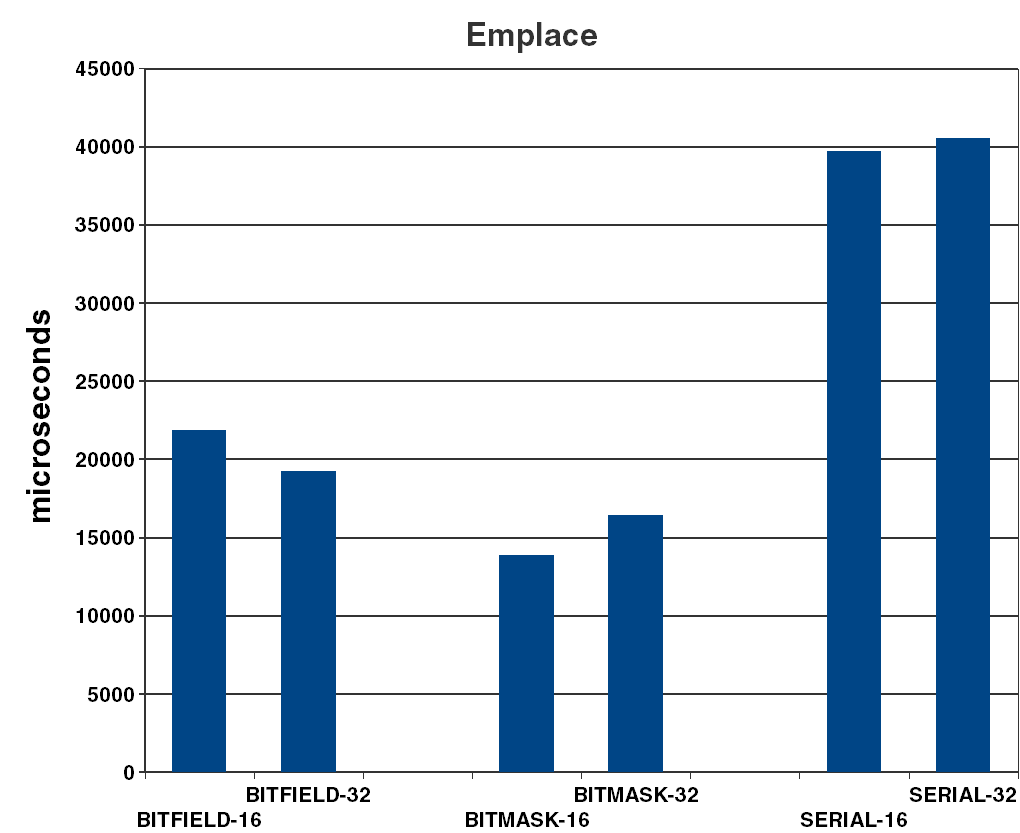Figure 1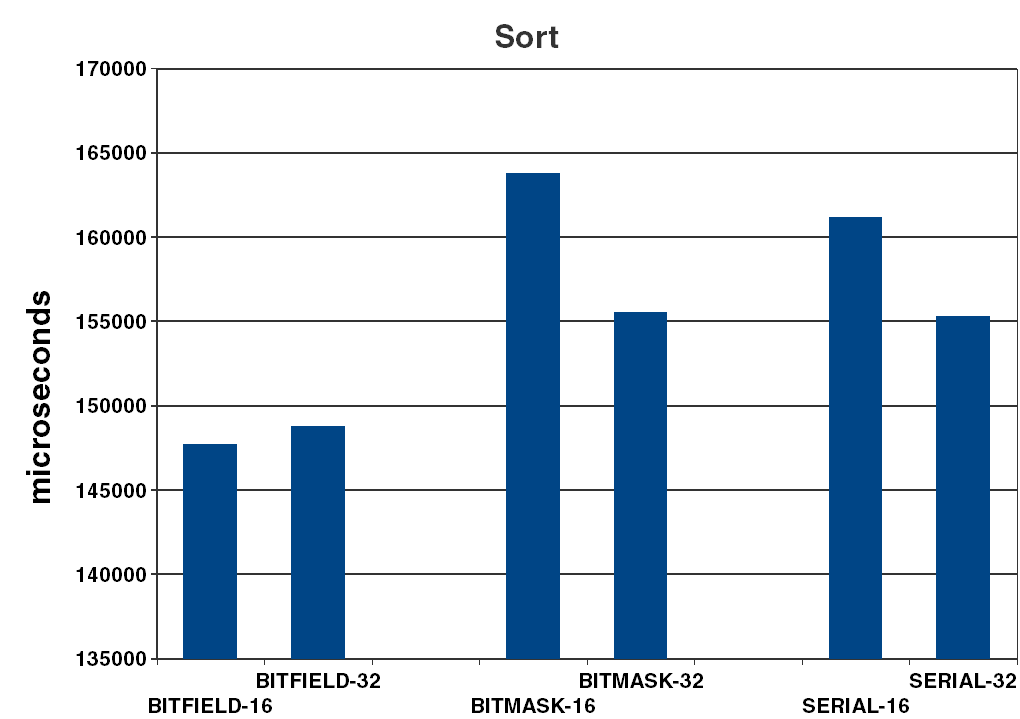Figure 2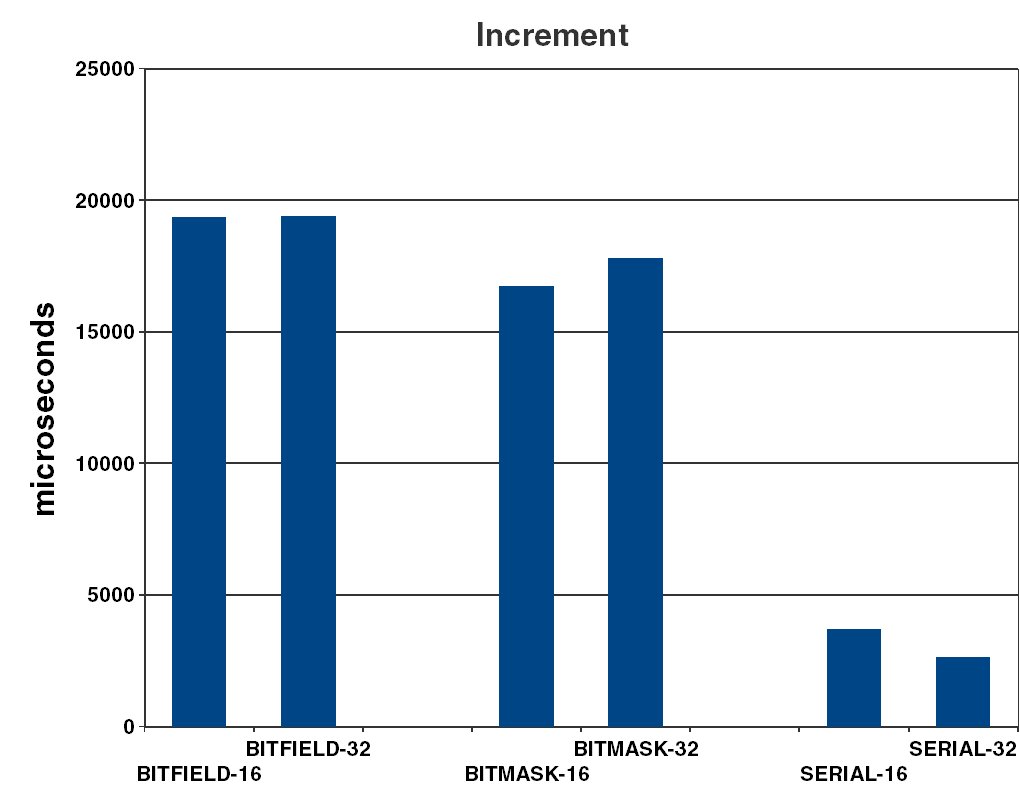Figure 3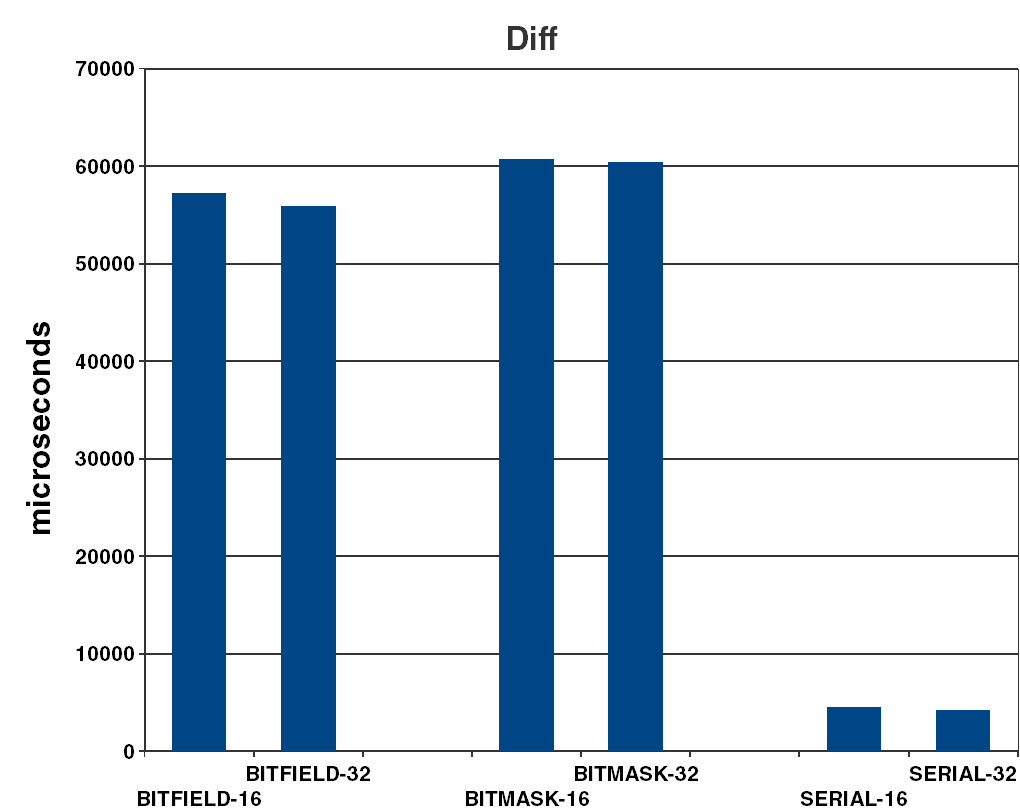Figure 4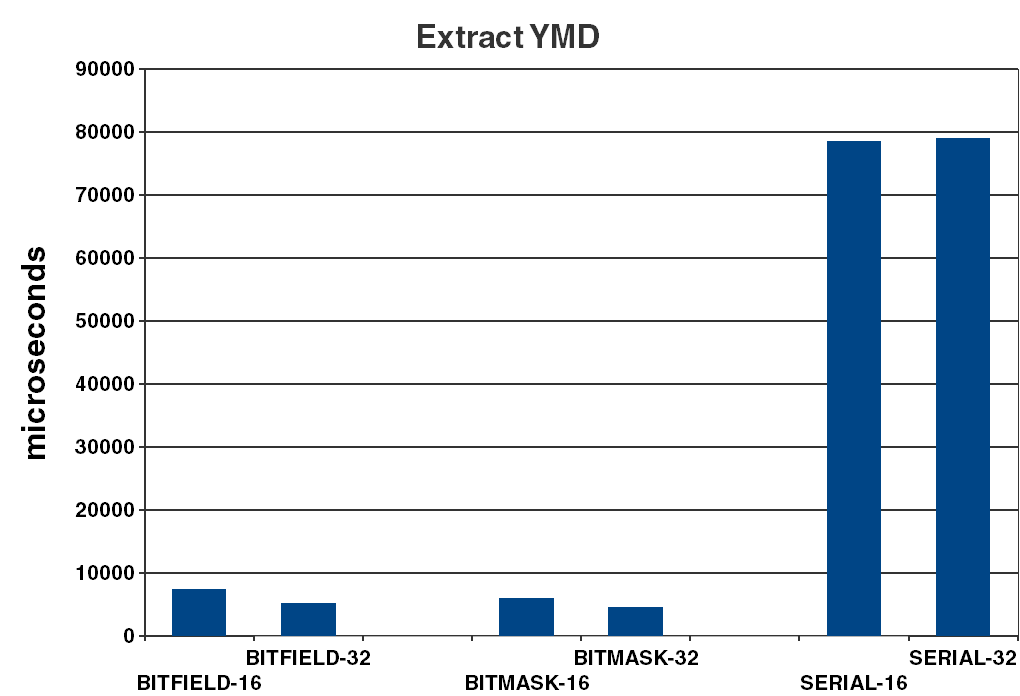Figure 5

All measurements are made on an old Samsung NC10 Netbook, running 32-bit Ubuntu 12.04, since it has the smallest cache of all computers I have readily available. The test programs are compiled with g++ 4.6.3 using ``` -O3 -std=c++0x ``` . The graphs are the averages of 100 runs of each measurement.

## Results

Almost all measurements show a performance advantage for the 32-bit representations, despite a lower number of cache misses (as shown by the valgrind tool ‘cachegrind’ [ cachegrind ].) This surprising result is consistent across a 4 different computers tested, all with different versions of x86 CPUs and also with a few different versions of g++.

## Conclusions

It is not surprising that there probably is no one representation that is best for every need.

For me, the noticeably better performance of hand written bit field management using mask/shift operations was a surprise, but it was understood once the code generated by the compiler was examined. This does not explain why the bitfield version was faster in some of the tests, though. Most peculiar is the substantial performance difference when sorting dates.

It definitely came as a surprise that cutting the size of the data set in half by using 16-bit representations almost always made a difference for the worse. Certainly there is a disadvantage for emplace and extract ymd, and on diff for the field based versions, since the epoch must be subtracted or added, but for all other measurements there is an obvious reduction in number of cache misses, and the increase in number of instructions or number of data accesses are insignificant, and still performance suffers a lot. Indeed, this find was so surprising that a CPU architecture buff of a colleague immediately asked for the source code to analyse.